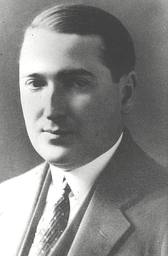• 1897 February 11
(b.) -
1954 April 21
(d.)

#### Bio/Description

In his doctoral thesis, Post proved, among other things, that the propositional calculus of Principia Mathematica was complete: all tautologies are theorems, given the Principia axioms and the rules of substitution and modus ponens. Post also devised truth tables independently of Wittgenstein and C.S. Peirce and put them to good mathematical use. Jean Van Heijenoort's well-known source book on mathematical logic (1966) reprinted Post's classic article setting out these results. In 1936, Post developed, independently of Alan Turing, a mathematical model of computation that was essentially equivalent to the Turing machine model. Intending this as the first of a series of models of equivalent power but increasing complexity, he titled his paper Formulation 1. (This model is sometimes called "Post's machine" or a Post-Turing machine, but is not to be confused with Post's tag machines or other special kinds of Post canonical system, a computational model using string rewriting and developed by Post in the 1920s but first published in 1943).
• Date of Birth:

1897 February 11
• Date of Death:

1954 April 21
• Gender:

Male
• Noted For:

Best known for his work in the field that eventually became known as computability theory
• Category of Achievement: